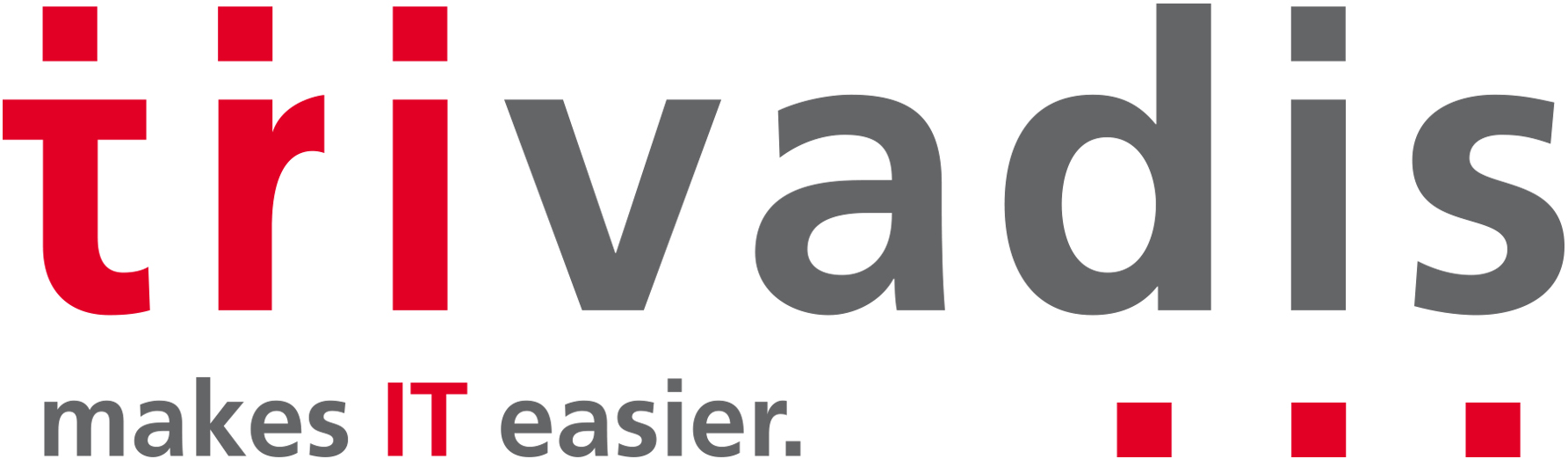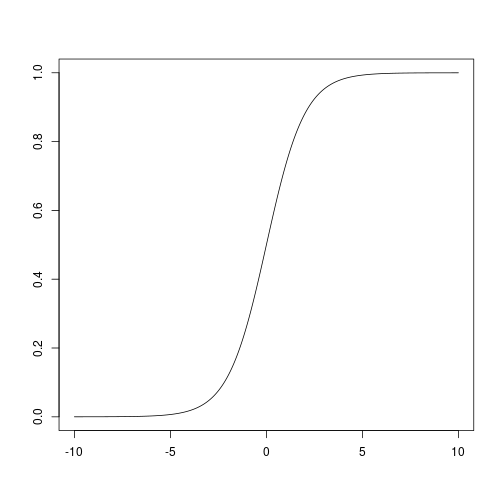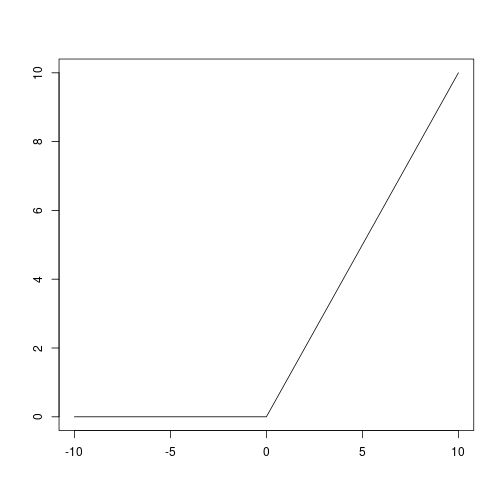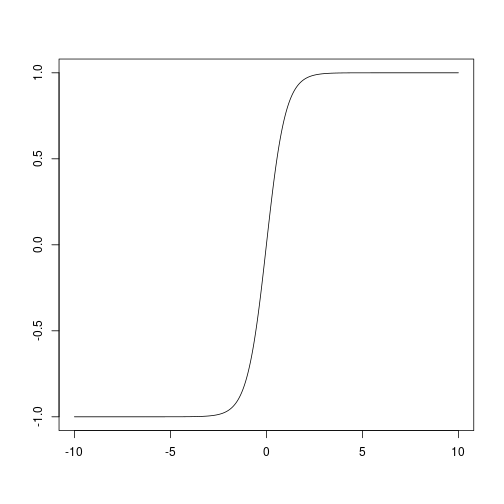# Deep learning, concepts and frameworks: Find your way through the jungle (Part 2)

02/06/2018

This is part 2, please see part 1 first :-)

# Depth alone is not enough: Activation functions

### Depth alone is not enoughHow ever deep we make our network, if we just chain layers of matrix multiplication one after another, all we get is a linear combination of the inputs.

How can we solve non-linear problems with neural networks?

### The classic: sigmoid activation function``````sigmoid <- function(x) 1/(1 + exp(-x))
x <- seq(-10,10, by = 0.01)
plot(x, sigmoid(x), type = "l", xlab = "", ylab = "")
``````### The current default, kind of: ReLU``````relu <- function(x){
x[x<0] <- 0
x
}
x <- seq(-10,10, by = 0.01)
plot(x, relu(x), type = "l", xlab = "", ylab = "")
``````### Mostly for LSTMs: tanh``````x <- seq(-10,10, by = 0.01)
plot(x, tanh(x), type = "l", xlab = "", ylab = "")
``````# Convolutional Neural Networks for Computer Vision

### Going spatial: ConvnetsLeNet: First successful application of convolutional neural networks by Yann LeCun, Yoshua Bengio et al.